# Simple Version of the Chain Rule

Suppose we want to find a derivative for this function:  h(x) = sin(2x).

The sine function is on our list – we know that the derivative of sin(x) is cos(x).  But we are not dealing with just the sine function.  There’s another function inside the function!  We don’t just have “sine of x” but rather we have “sine of something”, where the “something” is itself a function.

This means that to find this kind of derivative, it is essential that you have a clear understanding of the composition of two functions.  You have to look at  h(x) = sin(2x) and see it as the composition of functions and you have to recognize the building blocks:

g(x) = 2x and f(x) = sin(x)

We are not going to just add them or multiply them.  Instead, we use the output of one of them as the input to the other. So we build:

h(x) = f(g(x)) = sin(2x)

The g function is the inner function.  It starts off by doubling its input.  Then that output is handed off to the “outer function”,  f  which finds the sine.

And now, it we want to find the derivative, we need to learn…

THE CHAIN RULE (maybe)

Once again, I have to pause and remind myself of the purpose of these posts. If I show you  “the chain rule”, we can then use it to start finding derivatives of long, challenging “chains” of compositions of multiple functions, such as:But then I remember two things:

1. This is beyond what we will actually need in the opening months of AP Physics.

2. Your math teacher deserves to have some of the fun as well.

So I am only going to introduce a lesser version of the chain rule, one that is just enough to handle a special case, one that we will actually need in class very early in the year.

THE SIMPLE CHAIN RULE FOR CONSTANT MULTIPLIERS

For example, look at each of these: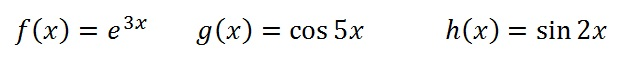In each case, the “outer” function is one from our list of well-known functions.  And the “inner” function is a constant multiplier.

There is an easy rule that handles all of these: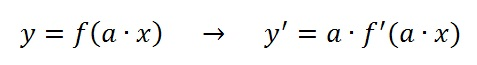In other words, go ahead and take the derivative using the familiar rule, but then multiply your answer by the constant you see “inside” the original expression.

With this rule we can find all three of the derivatives above: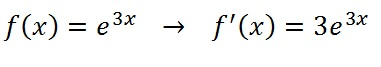The derivative of “e to the something” is “e to that something”, and the 3 is a multiplier.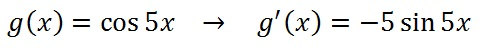The derivative of “the cosine of something” is “the minus sine of that something” and the 5 is a multiplier.The derivative of “the sine of something” is “the cosine of something” and the 2 is a multiplier.

DON’T JUST TAKE MY WORD FOR IT…

Why do we multiply by that same number you see in the inner function?  It turns out that there is an intuitive way to understand this rule.  Once again, we have to look at graphs and their transformations.

In the last post, we saw that compared to the graph of y = f(x), the graph of y = f(x) was stretched vertically when a>1, and that the vertical stretch affects the tangent line as well, also increasing its slope by that same factor, a.

But now we consider the graph of y = f(a·x), we see that a>1 leads to a horizontal compression, and that compression affects the tangent line as well.  Slope is rise over run, so reducing the run increases the slope, again by that same factor, a.  That’s why our derivatives all have that multiplier: compressing the graphs horizontally makes the slopes steeper.

For example, here are graphs of f(x) = ex and f(x) = e3x.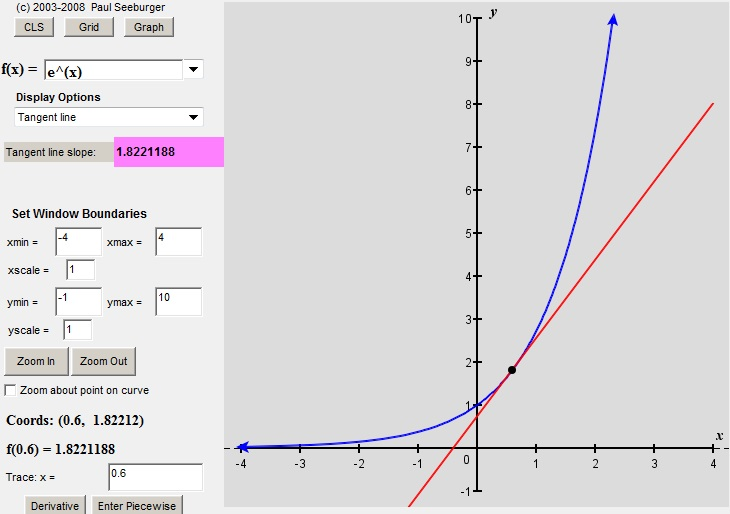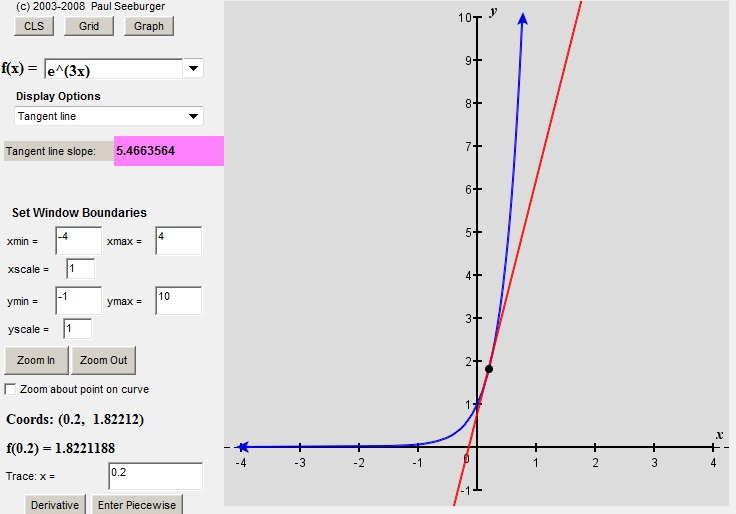You can see that the graph is horizontally compressed.

And when you compare the slopes of the tangent lines, you see that the second one is 3 times as steep as the first. [But notice: the first tangent line is at x=.6 and the second one is at x=.2.  Why did I do that?  Post a comment with your answer…]  Until your math teacher unveils the chain rule in its full glory, please let the diagram above serve as a proof of our simpler version. But in the mean time…

Here is an animated version of what I am trying to say:

And if you want to play with the Desmos file, it’s here.

SO WHERE ARE WE NOW?

At this point, we have assembled some basic derivative formulas and some rules about combining them.  This would be a good place to pause and see if you are keeping up.  So in the next post, you will find a set of practice questions.  Once you have mastered them, you will be ready to find out what we do with these slope-finding formulas once we have derived them.